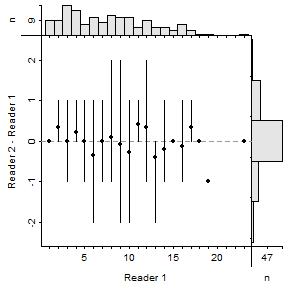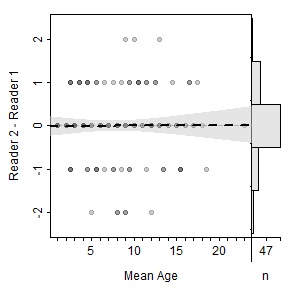# Age Bias Plot Changes in FSA

April 25, 2017
By

Want to share your content on R-bloggers? click here if you have a blog, or here if you don't.

In the last two weeks, I have posted twice about modifying age bias plots and Bland-Altman-like plots for comparing age estimates. From those posts, I have decided that I prefer to

• plot differences between the ages on the y-axis (as compared to the nonreference ages),
• plot overlapping points with a transparent color that becomes darker as more points overlap,
• when individual points are shown, plot a generalized additive model (GAM) that describes the relationship between the difference in ages and the reference ages or the mean of the two ages (if a reference age is not declared), and
• when individual data points are not shown, show the mean and range (rather than confidence intervals) of differences in ages at each reference age with open points representing means where a significant difference between the estimates is evident.

I recently updated the FSA package so that these preferences are the defaults, while still allowing users some flexibility in creating plots that fit their preferences. Here I explain this new functionality.

The functionality described here is available in the current development version of FSA and will eventually (during summer) be on CRAN as version 0.8.13. I welcome any comments or suggestions.

The data used here will again be ages of Lake Whitefish (Coregonus clupeaformis) from Lake Champlain that are available in the `WhitefishLC` data.frame in FSA. These analyses will compare consensus (between two readers) otolith (`otolithC`) and scale (`scaleC`) age estimates and otolith ages between two readers (`otolith1` and `otolith2`). The consensus otolith age estimates and otolith age estimates from the first reader will be considered as “reference” ages when such a distinction is needed.

# Age comparisons with summary statistics

The default plot of an `ageBias()` object is a modified age bias plot with the difference in age estimates on the y-axis, the reference age estimates on the x-axis, a reference line at a difference in age estimates of zero, the mean and the range of differences in age estimates shown for each reference age estimate, open points representing age estimates where the mean difference in age estimates is significantly different from zero, solid points representing age estimates where the mean difference in age estimates is not significantly different from zero, a marginal histogram at the top that shows the distribution (and sample sizes) of the reference age estimates, and a marginal histogram on the right that shows the distribution of the difference in age estimates. Confidence intervals for the mean differences in age estimates at each reference age estimate may be added with `show.CI=TRUE` and individual points can be added with `show.pts=TRUE`. Other options are described in the `ageBias()` documentation, which includes a number of examples.

The example in Figure 1 shows that age estimates from scales are less than age estimates from otoliths for otolith age estimates greater than about age-6 or 8, though the statistical evidence is less clear at older ages due to low sample sizes and increased variability. The example in Figure 2 illustrates no systematic difference in age estimates from otoliths between two readers. [Note that the y-axis limits here were widened from the defaults so that the bars in the marginal histogram were not cut off.]Figure 1: Mean (points) and range (intervals) of differences in scale and otolith age estimates at each otolith age estimate for Lake Champlain Lake Whitefish. Open points represent mean differences in scale and otolith age estimates that are significantly different from zero (dashed gray horizontal line). Marginal histograms are for otolith age estimates (top) and differences in scale and otolith age estimates (right).Figure 2: Mean (points) and range (intervals) of differences in otolith age estimates between two readers at the estimates for the first reader for Lake Champlain Lake Whitefish. Open points represent mean differences in age estimates that are significantly different from zero (dashed gray horizontal line). Marginal histograms are for age estimates of the first reader (top) and differences in age estimates between readers (right).

# Individual points with a GAM smoother

As discussed in this post, differences between two sets of age estimates can be revealed by plotting individual points with a summary for the relationship between the differences in age estimates and the reference or mean age estimates (whichever is used on the x-axis). These examples show how to create a base plot to which a summary can be added. These examples use the mean of the two age estimates on the x-axis, but the plot from the previous section with the reference age estimates on the x-axis could be used (but with `show.pts=TRUE` to show the individual points and `show.range=FALSE` to remove the mean and range intervals).

Before making the first example plot, a GAM will be fit to the differences and mean age estimates data. These data are contained in the `diff` and `mean` variables in the `data` object returned in the `ageBias()` object.

As shown in this post, the GAM is fit with `gam()` using `s()` from the `mgcv` package. The mean predicted differences in age estimates, and their standard errors, throughout the range of observed mean age estimates are calculated with `predict()` using `type="response"` and `se=TRUE`. Approximate 95% confidence intervals for the predicted mean differences in age estimates are computed from normal theory. The code below fits the GAM, creates a vector of mean age estimates at which to make predictions, makes the predictions, and computes the approximate 95% confidence intervals.

The base plot of individual differences in age estimates plotted against the mean age estimates is constructed by adding `xvals="mean"` to `plot()`. By default, a histogram for the difference in age estimates is shown on the right. A histogram for the mean age estimates is not shown by default but can be added at the top with `xHist=TRUE`. The `allowAdd=TRUE` argument is used so that “items”, like the GAM results, can be added to the main plot (i.e., not the marginal histograms). Note that using `allowAdd=TRUE` changes the current graphing parameters and that it is good practice to save the current graphing parameters (the first line below) so that they can be reset after finishing the plot (use of `par(op)` below).

The GAM results (line at the the predicted means and polygon for the 95% confidence bands) are then added to this plot as described in this post.

Finally, the graphing parameters are returned to their original values.

The example in Figure 3 suggests that the two age estimates generally agree to a mean age of about 5, after which ages estimated from scales are less than ages estimated from otoliths. The example in Figure 4 suggests no difference in age estimates between the two readers for all mean ages.Figure 3: Differences in scale and otolith age estimates at each mean age estimate for Lake Champlain Lake Whitefish. The dashed gray horizontal line is at 0, which represents no difference between scale and otolith age estimates. The dashed black line and gray polygon represent the mean and 95% confidence band for the predicted mean difference in age estimates from a generalized additive model. The right marginal histogram is for the differences in scale and otolith age estimates.Figure 4: Differences in otolith age estimates between two readers at each mean age estimate for Lake Champlain Lake Whitefish. The dashed gray horizontal line is at 0, which represents no difference in age estimates between the two readers. The dashed black line and gray polygon represent the mean and 95% confidence band for the predicted mean difference in age estimates from a generalized additive model. The right marginal histogram is for the differences in age estimates between the two readers.

My modification of the traditional age bias plot of Campana et al. (1995) is constructed from the `ageBias()` object with `plotAB()` (Figure 5). Some simple modifications of this plot are demonstrated in the documentation and examples for `plotAB()`.Figure 5: Mean (points) and 95% confidence intervals of scale age estimates at each otolith age estimate for Lake Champlain Lake Whitefish. The dashed gray line represents age estimates that agree. Open points (with red confidence intervals) represent mean scale age estimates that differ significantly from the corresponding otolith age estimate.

Finally, some users prefer a simple plot that shows the number of individuals at each point (Figure 6). This plot is constructed with `plotAB()` using `what="nunbers"`.Figure 6: Number of individuals by each scale and otolith age estimate combination for Lake Champlain Lake Whitefish. The dashed gray line represents age estimates that agree.

• Campana, S.E., M.C. Annand, and J.I. McMillan. 1995. Graphical and statistical methods for determining the consistency of age determinations. Transactions of the American Fisheries Society 124:131-138.

• Ogle, D.H. 2015. Introductory Fisheries Analyses with R book. CRC Press.

R-bloggers.com offers daily e-mail updates about R news and tutorials about learning R and many other topics. Click here if you're looking to post or find an R/data-science job.
Want to share your content on R-bloggers? click here if you have a blog, or here if you don't.